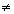CryptoSys Home > Triple DES cryptography software

# Triple DES cryptography software

Triple DES (3DES) or the Triple Data Encryption Algorithm (TDEA) is a refinement of the original Data Encryption Standard (DES).

Triple DES is described in NIST Special Publication 800-67. The original specification is in ANSI X9.52.

TDEA involves using three 64-bit DEA keys (K1, K2, K3) in Encrypt-Decrypt-Encrypt (EDE) mode, that is, the plain text is encrypted with K1, then decrypted with K2, and then encrypted again with K3. You will sometimes see this referred to as des-ede mode.

A TDEA key thus consists of three keys (K1, K2, K3). The three keys are also referred to as a key bundle. The key bundle is 3 x 64 = 192 bits long.

ANSI X9.52 describes three options for the selection of keys in a bundle. Option 1, the preferred option, employs three mutually independent keys (K1K2K3K1). Option 2 employs two mutually independent keys and a third key that is the same as the first key (K1K2 and K3 = K1). Option 3 is a key bundle of three identical keys (K1 = K2 = K3). Option 1 gives a keyspace of 3 x 56 = 168 bits.

## I have a triple DES key. What are its sub-keys?

Just split the 192-bit triple DES key into 3 separate 64-bit keys, working from left to right. For example, if your triple-DES key is the 192-bit value (in hex format):

```0123456789ABCDEFFEDCBA987654321089ABCDEF01234567
```

then split it into the three sub-keys, K1, K2 and K3, each of 64 bits:

``` 0123456789ABCDEF FEDCBA9876543210 89ABCDEF01234567
|<------K1------>|<------K2------>|<------K3------>|
```

So the sub-keys are K1=`0x0123456789ABCDEF`, K2=`0xFEDCBA9876543210` and K3=`0x89ABCDEF01234567`.

## Can I do single-key DES encryption with a Triple DES function?

If you encrypt something, then decrypt it and encrypt it again with the same key, you've just done the same as encrypting it once. Thus option 3 with three identical keys is simply the original 64-bit DEA algorithm with a keyspace of 56 bits, albeit done with three times as much effort. So, to carry out "single" DES using a Triple DES function, just set all three DEA keys to be the same. Note that this option is no longer permitted under NIST SP 800-67.

For example, if your single-DES key is `0x89ABCDEF01234567` then set your Triple-DES key to be

``` 89ABCDEF01234567 89ABCDEF01234567 89ABCDEF01234567
|<------K1------>|<------K2------>|<------K3------>|
```

## What's two-key triple DES encryption?

Two-key triple DES is option 2 where we encrypt with K1, then decrypt with K2 and finally encrypt again with K1. The keyspace is thus 2 x 56 = 112 bits.

For example, with K1=`0x0123456789ABCDEF` and K2=`0xFEDCBA9876543210` you would set the triple DES key to be `0x0123456789ABCDEFFEDCBA98765432100123456789ABCDEF`.

``` 0123456789ABCDEF FEDCBA9876543210 0123456789ABCDEF
|<------K1------>|<------K2------>|<------K3------>|
```

## How long is a triple DES key: 192 or 168 bits?

As mentioned above, a triple DES key is a bundle of three DES keys. A DES key is 64 bits long, but only 56 bits of these are used in the encryption process.

A triple DES key is therefore 3 x 64 = 192 bits long, but the keyspace is only 3 x 56 = 168 bits.

Note that if your "triple DES" key is not exactly 192-bits long - i.e. exactly 24 bytes long, or 48 hexadecimal digits - then you do not have a valid triple DES key.

The other 8 bits are meant to be used as error detecting or "parity" bits so, in principle, the validity of the key bit string can be checked (every byte should be of odd parity), but this is hardly ever done. Most people and most software packages, including CryptoSys API and CryptoSys PKI, do not bother to check the parity of the key and just ignore the state of the parity bits. Be careful, though, because this means that the keys represented, for example, by the following bit strings are treated as being equal, and will produce identical ciphertext output for the same plaintext input:

```0123456789ABCDEF FEDCBA9876543210 89ABCDEF01234567
0022446688AACCEE FFDDBB9977553311 88AACCEE00224466
```

## Modes of operation

X9.52 describes seven different modes for using TDEA. CryptoSys API and the CryptoSys PKI Toolkit support the five most useful of these: Electronic Codebook (ECB), Cipher Block Chaining (CBC), 64-bit Cipher Feedback mode (CFB), Output Feedback mode (OFB) and Counter mode (CTR).

## Triple DES functions available in CryptoSys API

### Recommended functions and methods

We recommend you use the CIPHER functions and Cipher methods with the tdea option.

### Original Triple DES-specific functions (deprecated)

The equivalent .NET methods are in the Tdea Class.